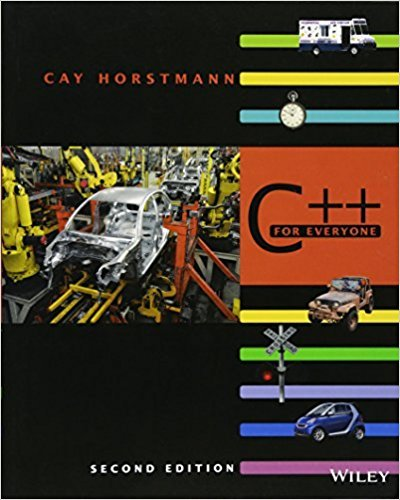×
Get Full Access to C++ For Everyone - 2 Edition - Chapter 2 - Problem P2.16
Get Full Access to C++ For Everyone - 2 Edition - Chapter 2 - Problem P2.16

×

# File names and extensions. Write a program that prompts the user for the driveISBN: 9780470927137 356

## Solution for problem P2.16 Chapter 2

C++ for Everyone | 2nd Edition

• Textbook Solutions
• 2901 Step-by-step solutions solved by professors and subject experts
• Get 24/7 help from StudySoup virtual teaching assistantsC++ for Everyone | 2nd Edition

4 5 1 259 Reviews
24
2
Problem P2.16

File names and extensions. Write a program that prompts the user for the drive letter(C), the path (\Windows\System), the file name (Readme), and the extension (txt). Thenprint the complete file name C:\Windows\System\Readme.txt. (If you use UNIX or aMacintosh, skip the drive name and use / instead of \ to separate directories.)

Step-by-Step Solution:
Step 1 of 3

MODULE 4 ARTICLE I. INFERENCE FOR POPULATION MEANS 1) Confidence Intervals a. We can find confidence intervals of two differencesample means 2) Known Population Variances a. Let X and Y be to independent binomial random variables. b. A 100(1-α)% CI for the difference of the two meansfrom each random variable is: 3) Unknown population variance a. t-value is used 4) Large sample confidence intervals for two population proportions a. Let X and Y be to independent binomial random variables. b. Proportion p is the number of successes/n ̃ c. Let n =nx+2x d. Let p =(x+1)/ n x e. The CI is therefore: Note: the difference of two proportions should liebetween -1 and 1 5) Paired Data a. There are instances when two samples are not independent, this is when the data is paired. b. It is referred to as paired because we consider thedata in pairs, (X ,Y ) 1 1 c. We use the data set of differences, D =X -Y fo1 ca1cul1tion i. This reduces a 2 sample problem to a 1 sample problem d. This holds equivalently for the means of two separate samples e. NOTE: differences (D) can be incorporated into covariance by:

Step 2 of 3

Step 3 of 3

## Discover and learn what students are asking

Chemistry: The Central Science : Molecular Geometry and Bonding Theories
?In the hydrocarbon (a) What is the hybridization at each carbon atom in the molecule? (b) How many \(

Statistics: Informed Decisions Using Data : Inference about Measures of Central Tendency
?Write a paragraph that describes the logic of the test statistic in a right-tailed sign test.

Unlock Textbook Solution